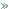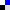HomeDesign PrinciplesLiskov's Substitution PrincipleBookmark this on Delicious

# Liskov's Substitution Principle(LSP)

## Motivation

All the time we design a program module and we create some class hierarchies. Then we extend some classes creating some derived classes.

We must make sure that the new derived classes just extend without replacing the functionality of old classes. Otherwise the new classes can produce undesired effects when they are used in existing program modules.

Likov's Substitution Principle states that if a program module is using a Base class, then the reference to the Base class can be replaced with a Derived class without affecting the functionality of the program module.

## Intent

Derived types must be completely substitutable for their base types.

## Example

Below is the classic example for which the Likov's Substitution Principle is violated. In the example 2 classes are used: Rectangle and Square. Let's assume that the Rectangle object is used somewhere in the application. We extend the application and add the Square class. The square class is returned by a factory pattern, based on some conditions and we don't know the exact what type of object will be returned. But we know it's a Rectangle. We get the rectangle object, set the width to 5 and height to 10 and get the area. For a rectangle with width 5 and height 10 the area should be 50. Instead the result will be 100

 ```// Violation of Likov's Substitution Principle class Rectangle { protected int m_width; protected int m_height; public void setWidth(int width){ m_width = width; } public void setHeight(int height){ m_height = height; } public int getWidth(){ return m_width; } public int getHeight(){ return m_height; } public int getArea(){ return m_width * m_height; } } class Square extends Rectangle { public void setWidth(int width){ m_width = width; m_height = width; } public void setHeight(int height){ m_width = height; m_height = height; } } class LspTest { private static Rectangle getNewRectangle() { // it can be an object returned by some factory ... return new Square(); } public static void main (String args[]) { Rectangle r = LspTest.getNewRectangle(); r.setWidth(5); r.setHeight(10); // user knows that r it's a rectangle. // It assumes that he's able to set the width and height as for the base class System.out.println(r.getArea()); // now he's surprised to see that the area is 100 instead of 50. } } ```

## Conclusion

This principle is just an extension of the Open Close Principle and it means that we must make sure that new derived classes are extending the base classes without changing their behavior.